Physical constant facts for kids

Kids Encyclopedia Facts

In science, a physical constant is a number (physical quantity) that does not change. This is different from a mathematical constant, which is not based on a real measurement.

There are many physical constants in science. Some famous ones are the speed of light, Planck's constant, the gravitational constant, and Avogadro's number.

Some scientists think that physical constants may decrease as the universe gets older. There has been no evidence to support this yet.

Constants that do not have units (like length or speed) are called fundamental physical constants.

Some believe that if the physical constants were different, there would be no life in the universe. They say that the universe seems to be fine-tuned for intelligent life.

Table of physical constants

Quantity Symbol Value Relative Standard Uncertainty
speed of light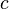$c \,$ 299 792 458 m·s−1 defined
constant of gravity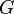$G \,$ 6.67428(67) × 10−11m³·kg−1·s−2 1.0 × 10−4
Planck's constant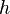$h \,$ 6.626 068 96(33) × 10−34 J·s 5.0 × 10−8
smallest electrical charge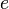$e \,$ 1.602 176 487(40) × 10−19 C 2.5 × 10−8
electron mass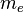$m_e \,$ 9.109 382 15(45) × 10−31 kg 5.0 × 10−8

Related pagesPhysical constant Facts for Kids. Kiddle Encyclopedia.